# 一文讲透“进程，线程和协程”

• 什么是进程，线程和协程？
• 它们之间的关系是什么？
• 为什么说Python中的多线程是伪多线程？
• 不同的应用场景该如何选择技术方案？
• ...

### 什么是进程

• 初始状态：进程刚被创建，由于其他进程正占有CPU资源，所以得不到执行，只能处于初始状态。
• 就绪状态：只有处于就绪状态的经过调度才能到执行状态
• 等待状态：进程等待某件事件完成
• 执行状态：任意时刻处于执行状态的进程只能有一个（对于单核CPU来讲）。
• 停止状态：进程结束

#### 进程间的切换

• 单核CPU双进程的情况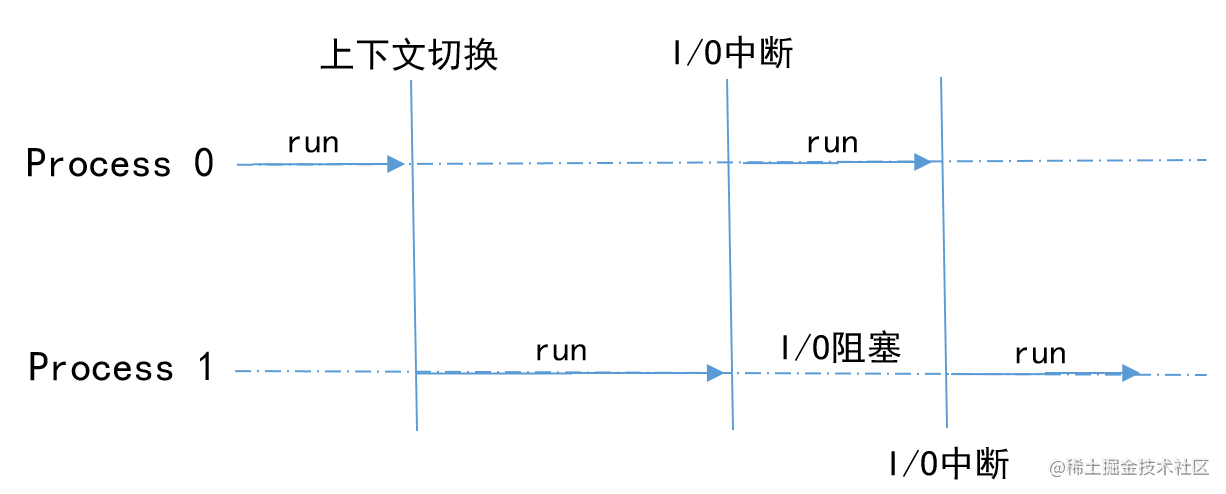• 双核CPU双进程的情况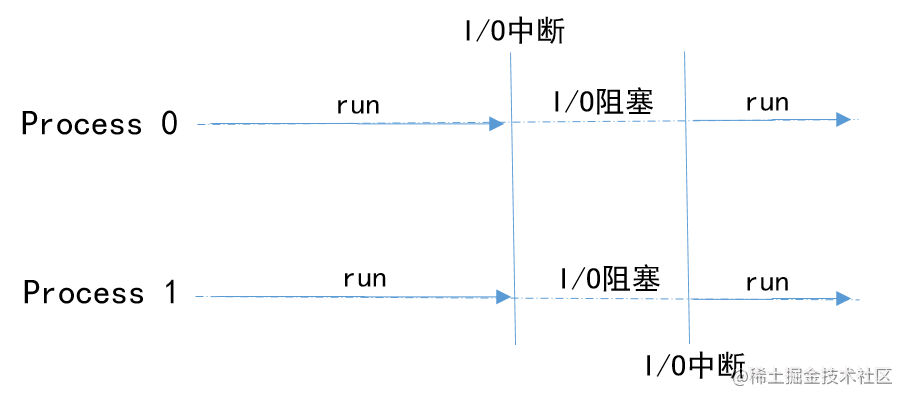#### 进程间数据共享

• 将主存看成是一个存储在磁盘上的高速缓存，在主存中只保存活动区域，并根据需要在磁盘和主存之间来回传送数据，通过这种方式，更高效地使用主存
• 为每个进程提供一致的地址空间，从而简化存储器管理
• 保护每个进程的地址空间不被其他进程破坏

• 以python中多进程（multiprocessing）为例：
``````import multiprocessing
import time

n = 0

def count(num):
global n
for i in range(100000):
n += i
print("Process {0}:n={1},id(n)={2}".format(num, n, id(n)))

if __name__ == '__main__':
start_time = time.time()

process = list()
for i in range(5):
p = multiprocessing.Process(target=count, args=(i,)) # 测试多进程使用
# p = threading.Thread(target=count, args=(i,))  # 测试多线程使用
process.append(p)

for p in process:
p.start()

for p in process:
p.join()

print("Main:n={0},id(n)={1}".format(n, id(n)))
end_time = time.time()
print("Total time:{0}".format(end_time - start_time))

• 结果
``````Process 1:n=4999950000,id(n)=139854202072440
Process 0:n=4999950000,id(n)=139854329146064
Process 2:n=4999950000,id(n)=139854202072400
Process 4:n=4999950000,id(n)=139854201618960
Process 3:n=4999950000,id(n)=139854202069320
Main:n=0,id(n)=9462720
Total time:0.03138256072998047

### 什么是线程

• 线程标志符
• 一组寄存器
• 线程运行状态
• 优先级
• 线程专有存储区
• 信号屏蔽

#### 进程 VS 线程

• 进程是资源的分配和调度的独立单元。进程拥有完整的虚拟地址空间，当发生进程切换时，不同的进程拥有不同的虚拟地址空间。而同一进程的多个线程共享同一地址空间（不同进程之间的线程无法共享）
• 线程是CPU调度的基本单元，一个进程包含若干线程（至少一个线程）。
• 线程比进程小，基本上不拥有系统资源。线程的创建和销毁所需要的时间比进程小很多
• 由于线程之间能够共享地址空间，因此，需要考虑同步和互斥操作
• 一个线程的意外终止会影响整个进程的正常运行，但是一个进程的意外终止不会影响其他的进程的运行。因此，多进程程序安全性更高。

### 什么是协程

• 协程可以比作子程序，但执行过程中，子程序内部可中断，然后转而执行别的子程序，在适当的时候再返回来继续执行。协程之间的切换不需要涉及任何系统调用或任何阻塞调用
• 协程只在一个线程中执行，是子程序之间的切换，发生在用户态上。而且，线程的阻塞状态是由操作系统内核来完成，发生在内核态上，因此协程相比线程节省了线程创建和切换的开销
• 协程中不存在同时写变量冲突，因此，也就不需要用来守卫关键区块的同步性原语，比如互斥锁、信号量等，并且不需要来自操作系统的支持。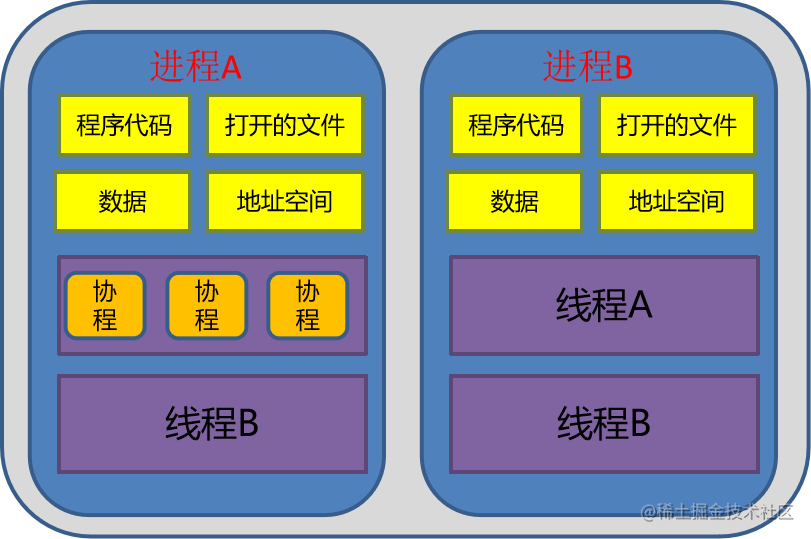### 如何选择？

``````Process 0:n=5756690257,id(n)=140103573185600
Process 2:n=10819616173,id(n)=140103573185600
Process 1:n=11829507727,id(n)=140103573185600
Process 4:n=17812587459,id(n)=140103573072912
Process 3:n=14424763612,id(n)=140103573185600
Main:n=17812587459,id(n)=140103573072912
Total time:0.1056210994720459

• n是全局变量，Main的打印结果与线程相等，证明了线程之间是数据共享

##### 什么是GIL

GIL来源于Python设计之初的考虑，为了数据安全(由于内存管理机制中采用引用计数)所做的决定。某个线程想要执行，必须先拿到 GIL。因此，可以把 GIL 看作是“通行证”,并且在一个 Python进程中，GIL 只有一个,拿不到通行证的线程,就不允许进入 CPU 执行。

Cpython解释器在内存管理中采用引用计数，当对象的引用次数为0时，会将对象当作垃圾进行回收。（有关Python内存管理机制的相关内容可以参见面试必备：Python内存管理机制）设想这样一种场景：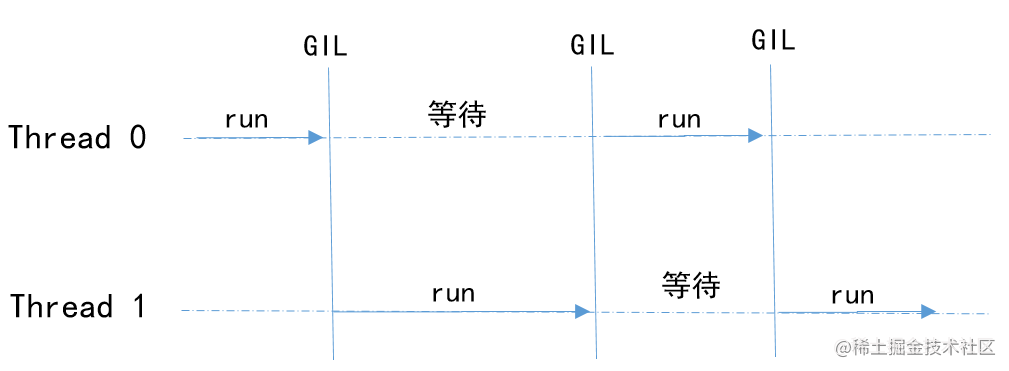#### 何时用？

• CPU密集型:程序需要占用CPU进行大量的运算和数据处理；
• I/O密集型:程序中需要频繁的进行I/O操作；例如网络中socket数据传输和读取等；
• CPU密集+I/O密集：以上两种的结合

##### 什么是DMA

DMA(Direct Memory Access)是系统中的一个特殊设备，它可以协调完成内存到设备间的数据传输，中间过程不需要CPU介入

• 进程p1发出数据写入磁盘文件的请求
• CPU处理写入请求，通过编程告诉DMA引擎数据在内存的位置，要写入数据的大小以及目标设备等信息
• CPU处理其他进程p2的请求，DMA负责将内存数据写入到设备中
• DMA完成数据传输，中断CPU
• CPU从p2上下文切换到p1,继续执行p1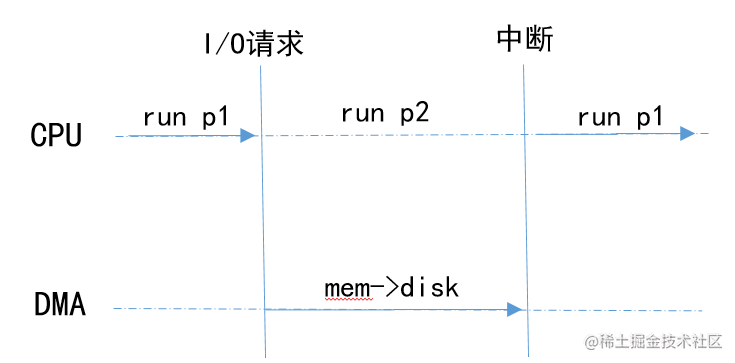##### Python多线程的表现（I/O密集型）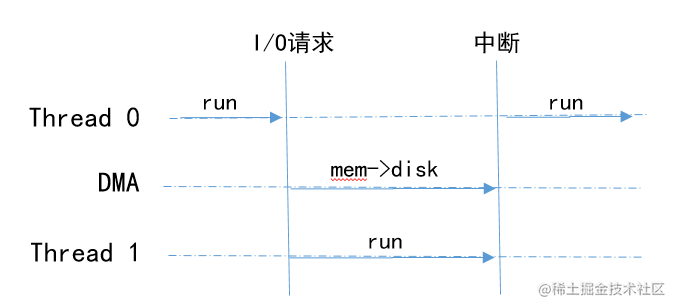##### 测试
• 执行代码
``````import multiprocessing
import time

def count(num):
time.sleep(1)  ## 模拟IO操作
print("Process {0} End".format(num))

if __name__ == '__main__':
start_time = time.time()
process = list()
for i in range(5):
p = multiprocessing.Process(target=count, args=(i,))
process.append(p)

for p in process:
p.start()

for p in process:
p.join()

end_time = time.time()
print("Total time:{0}".format(end_time - start_time))

• 结果
``````## 多进程
Process 0 End
Process 3 End
Process 4 End
Process 2 End
Process 1 End
Total time:1.383193016052246
## 多线程
Process 0 End
Process 4 End
Process 3 End
Process 1 End
Process 2 End
Total time:1.003425121307373

• 多线程的执行效性能高于多进程

• 程序代码
``````import time
import asyncio

async def coroutine():
await asyncio.sleep(1) ## 模拟IO操作

if __name__ == "__main__":
start_time = time.time()

loop = asyncio.get_event_loop()
for i in range(5):

loop.close()
end_time = time.time()
print("total time:", end_time - start_time)

• 结果
``````total time: 1.001854419708252

• 协程的执行效性能高于多线程

### 总结

• CPU密集型： 多进程
• IO密集型： 多线程（协程维护成本较高,而且在读写文件方面效率没有显著提升）
• CPU密集和IO密集： 多进程+协程